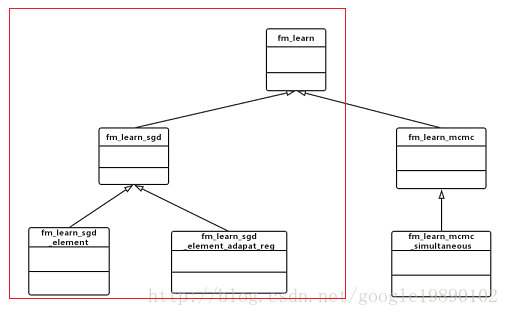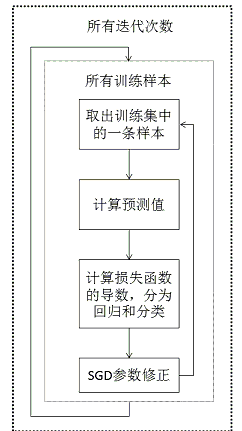# 5.1、基于梯度的模型训练方法fm_learn_sgd类是所有基于梯度的训练方法的父类，其具体的代码如下所示：

#include "fm_learn.h"
#include "../../fm_core/fm_sgd.h"

// 继承自fm_learn
class fm_learn_sgd: public fm_learn {
protected:
//DVector<double> sum, sum_sqr;
public:
int num_iter;// 迭代次数
double learn_rate;// 学习率
DVector<double> learn_rates;// 多个学习率

// 初始化
virtual void init() {
fm_learn::init();
learn_rates.setSize(3);// 设置学习率
//  sum.setSize(fm->num_factor);
//  sum_sqr.setSize(fm->num_factor);
}

// 利用梯度下降法进行更新，具体的训练的过程在其子类中
virtual void learn(Data& train, Data& test) {
fm_learn::learn(train, test);// 该函数并没有具体实现
// 输出运行时的参数，包括：学习率，迭代次数
std::cout << "learnrate=" << learn_rate << std::endl;
std::cout << "learnrates=" << learn_rates(0) << "," << learn_rates(1) << "," << learn_rates(2) << std::endl;
std::cout << "#iterations=" << num_iter << std::endl;

if (train.relation.dim > 0) {// 判断relation
throw "relations are not supported with SGD";
}
std::cout.flush();// 刷新
}

// SGD重新修正fm模型的权重
void SGD(sparse_row<DATA_FLOAT> &x, const double multiplier, DVector<double> &sum) {
fm_SGD(fm, learn_rate, x, multiplier, sum);// 调用fm_sgd中的fm_SGD函数
}

// debug函数，主要用于打印中间结果
void debug() {
std::cout << "num_iter=" << num_iter << std::endl;
fm_learn::debug();
}

// 对数据进行预测
virtual void predict(Data& data, DVector<double>& out) {
assert(data.data->getNumRows() == out.dim);// 判断样本个数是否相等
for (data.data->begin(); !data.data->end(); data.data->next()) {
double p = predict_case(data);// 得到线性项和交叉项的和，调用的是fm_learn中的方法
p = std::min(max_target, p);
p = std::max(min_target, p);
p = 1.0/(1.0 + exp(-p));// Sigmoid函数处理
} else {// 异常处理
}
out(data.data->getRowIndex()) = p;
}
}

};

fm_learn_sgd类中，主要包括五个函数，分别为：初始化init函数，训练learn函数，SGD训练SGD函数，debug的debug函数和预测predict函数。

## 5.1.2、训练learn函数

learn函数中，没有具体的训练的过程，只是对训练中需要用到的参数进行输出，具体的训练的过程在其对应的子类中定义，如fm_learn_sgd_element类和fm_learn_sgd_element_adapt_reg类。

## 5.1.3、SGD训练SGD函数

SGD函数使用的是fm_sgd.h文件中的fm_SGD函数。fm_SGD函数是利用梯度下降法对模型中的参数进行调整，以得到最终的模型中的参数。在利用梯度下降法对模型中的参数进行调整的过程中，假设损失函数为 l $l$，那么，对于回归问题来说，其损失函数为：

l=12(y^(i)y(i))2

l=lnσ(y^(i)y(i))

σ(x)=11+e(x)

σ=σ(1σ)

lθ=(y^(i)y(i))y^(i)θ

lθ=(σ(y^(i)y(i))1)y(i)y^(i)θ

lθ+λθ

y^:=w0+i=1nwixi+i=1n1j=i+1nvi,vjxixj

y^θ=1xixi(j=1xjvj,fxivi,f) if θ=w0 if θ=wi if θ=vi,f

θ=θη(lθ+λθ)

// 利用SGD更新模型的参数
void fm_SGD(fm_model* fm, const double& learn_rate, sparse_row<DATA_FLOAT> &x, const double multiplier, DVector<double> &sum) {
// 1、常数项的修正
if (fm->k0) {
double& w0 = fm->w0;
w0 -= learn_rate * (multiplier + fm->reg0 * w0);
}
// 2、一次项的修正
if (fm->k1) {
for (uint i = 0; i < x.size; i++) {
double& w = fm->w(x.data[i].id);
w -= learn_rate * (multiplier * x.data[i].value + fm->regw * w);
}
}
// 3、交叉项的修正
for (int f = 0; f < fm->num_factor; f++) {
for (uint i = 0; i < x.size; i++) {
double& v = fm->v(f,x.data[i].id);
double grad = sum(f) * x.data[i].value - v * x.data[i].value * x.data[i].value;
v -= learn_rate * (multiplier * grad + fm->regv * v);
}
}
}

## 5.1.4、预测predict函数

predict函数用于对样本进行预测，这里使用到了predict_case函数，该函数在“机器学习算法实现解析——libFM之libFM的训练过程概述”中有详细的说明，得到值后，分别对回归问题和分类问题做处理，在回归问题中，主要是防止超出最大值和最小值，在分类问题中，将其值放入Sigmoid函数，得到最终的结果。

# 5.2、SGD的训练方法#include "fm_learn_sgd.h"

// 继承了fm_learn_sgd
class fm_learn_sgd_element: public fm_learn_sgd {
public:
// 初始化
virtual void init() {
fm_learn_sgd::init();
// 日志输出
if (log != NULL) {
}
}
// 利用SGD训练FM模型
virtual void learn(Data& train, Data& test) {
fm_learn_sgd::learn(train, test);// 输出参数信息

std::cout << "SGD: DON'T FORGET TO SHUFFLE THE ROWS IN TRAINING DATA TO GET THE BEST RESULTS." << std::endl;
// SGD
for (int i = 0; i < num_iter; i++) {// 开始迭代，每一轮的迭代过程
double iteration_time = getusertime();// 记录开始的时间
for (train.data->begin(); !train.data->end(); train.data->next()) {// 对于每一个样本
double p = fm->predict(train.data->getRow(), sum, sum_sqr);// 得到样本的预测值
double mult = 0;// 损失函数的导数
if (task == 0) {// 回归
p = std::min(max_target, p);
p = std::max(min_target, p);
// loss=(y_ori-y_pre)^2
mult = -(train.target(train.data->getRowIndex())-p);// 对损失函数求导
} else if (task == 1) {// 分类
// loss
mult = -train.target(train.data->getRowIndex())*(1.0-1.0/(1.0+exp(-train.target(train.data->getRowIndex())*p)));
}
// 利用梯度下降法对参数进行学习
SGD(train.data->getRow(), mult, sum);
}
iteration_time = (getusertime() - iteration_time);// 记录时间差
// evaluate函数是调用的fm_learn类中的方法
double rmse_train = evaluate(train);// 对训练结果评估
double rmse_test = evaluate(test);// 将模型应用在测试数据上
std::cout << "#Iter=" << std::setw(3) << i << "\tTrain=" << rmse_train << "\tTest=" << rmse_test << std::endl;
// 日志输出
if (log != NULL) {
log->log("rmse_train", rmse_train);
log->log("time_learn", iteration_time);
log->newLine();
}
}
}

};

learn函数中，实现了SGD训练FM模型的主要过程，在实现的过程中，分别调用了SGD函数和evaluate函数，其中SGD函数如上面的5.1.3、SGD训练SGD函数小节所示，利用SGD函数对FM模型中的参数进行更新，evaluate函数如“机器学习算法实现解析——libFM之libFM的训练过程概述”中所示，evaluate函数用于评估学习出的模型的效果。其中mult变量分别对应着回归中的 (y^(i)y(i)) $\left ( \hat{y}^{(i)}-y^{(i)} \right )$和分类中的 (σ(y^(i)y(i))1)y(i) $\left ( \sigma \left ( \hat{y}^{(i)}y^{(i)} \right )-1 \right )\cdot y^{(i)}$

# 参考文献

• Rendle S. Factorization Machines[C]// IEEE International Conference on Data Mining. IEEE Computer Society, 2010:995-1000.
• Rendle S. Factorization Machines with libFM[M]. ACM, 2012.

04-221万+
11-301万+12-051万+
04-061万+
12-061377
11-103万+
04-144047
11-013278
08-076159
09-29201
10-082153
01-04166
05-187万+
01-052万+
09-204549